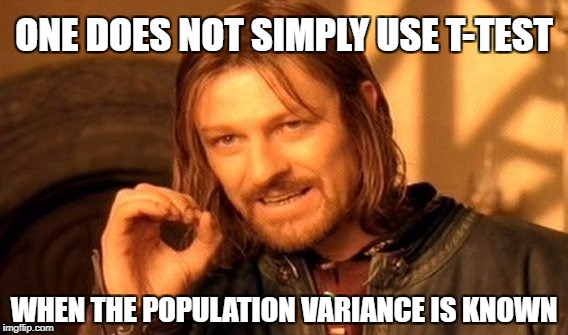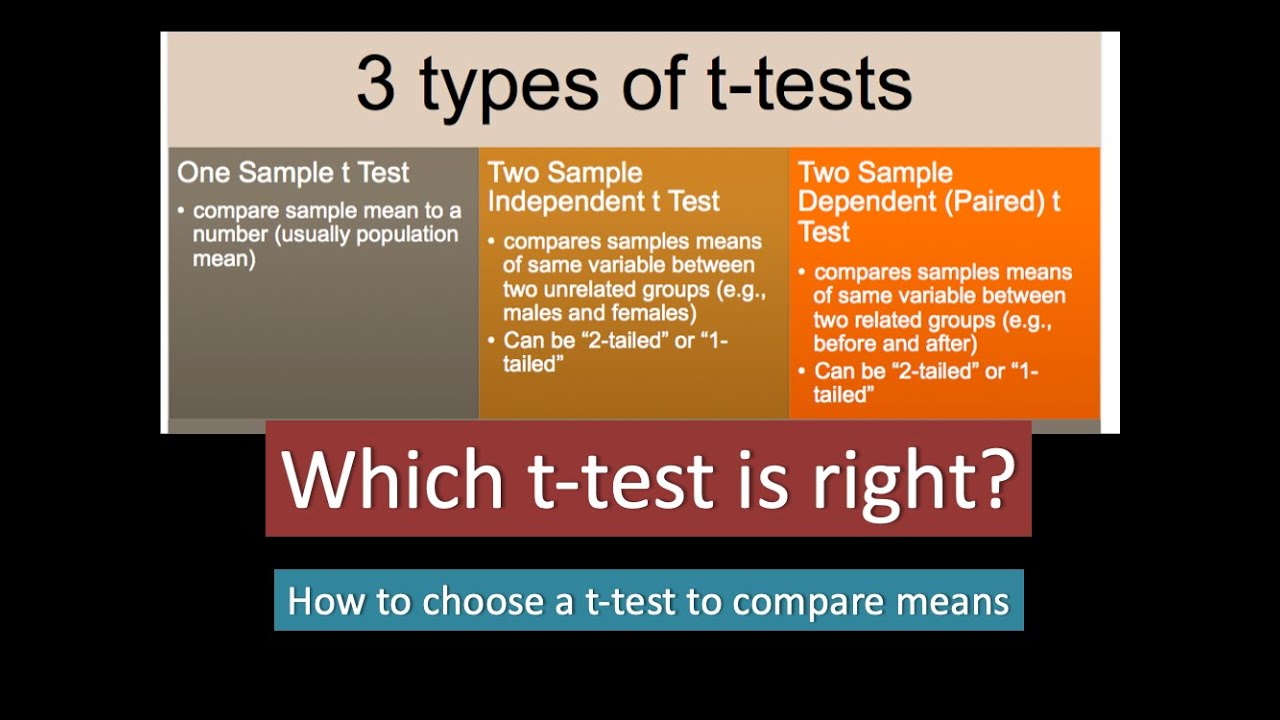# t-Tests

Published:

This post covers t-Tests.# t-Distribution

• Z - test works when we know $\mu$ and $\sigma$
• Use Samples
• How different a sample mean is from a population
• How different two sample means are from each other
• Two samples can be
• Independent
• Dependent
• Estimate Population Standard Deviation using sample standard deviation with Bessel’s correction
• Bessel’s correction is the use of $n − 1$ instead of $n$ in the formula for the sample variance and sample standard deviation, where $n$ is the number of observations in a sample.
• This method corrects the bias in the estimation of the population variance.
• It also partially corrects the bias in the estimation of the population standard deviation.
• However, the correction often increases the mean squared error in these estimations.
• This technique is named after Friedrich Bessel.
• To find out how typical or atypical (unusual) a sample mean - find its location on the distribution of sample means i.e. sampling distribution
• we can determine when we know population parameters, $\mu, \sigma$
• $std~errro= \frac{\sigma}{\sqrt{n}}$
• $z = \frac{sample~mean - \mu}{std~error} = \frac{mean~difference}{std~error}$
• Std for Samples = $S = \sqrt{\frac{\Sigma(X_i - \bar{X})^2}{n-1}}$
• Standard Error depends on sample, we cannot use $\sigma$ if we have sample
• Thus, we have a new distribution that is more prone to error - t-Distribution
• more spread out and thicker in the tails than a normal distribution
• Since large sample sizes gives skinnier sampling distribution
• What happens as n increases?
• The t-Distribution approaches to Normal Distribution
• The t-Distribution gets Skinnier tails
• $S \rightarrow \sigma$

# Degree of Freedom - Sample Standard Deviation

• We can pick a sample of size $n$ from population using $n$ degrees of freedom
• Now to compute Standard Deviation, we need sample mean
• $\bar{X} = \frac{X_1+X_2+X_3+…+X_n}{n}$
• $X_1+X_2+X_3+…+X_n = n . \bar{X}$
• $n-1$ Degrees of Freedom
• We may vary $n-1$ values to keep the sum of these values as $n\bar{X}$
• $n-1$ is the effective sample size since only $n-1$ values are independent if we know the mean.
• $S = \sqrt{\frac{\Sigma(X_i - \bar{X})^2}{n-1}}$
• As degrees of freedom increases, the t-distribution better approxiamate the normal distribution

# t-Table

• https://naneja.github.io/python/t-table
• Questions
1. What’s the t-critical value for a one-tailed alpha level of 0.05 with 12 degrees of freedom.

• df = 11 and p = 0.05
• Ans t = 1.782
2. What are t-critical values for 2-tailed test with $\alpha = 0.05$ and sample size 30

• $df = 29,~ p = \pm 0.025$
• Ans: $\pm 2.045$
3. What are the limits for the right area of t-statistic when the sample size is 24 and the t-statistic is 2.45

• $df=23, t=2.45$
• $p = .02 ~\text{or}~ .01$

# t-Statistic

$t = \frac{\bar{X}-\mu_0}{\frac{S}{\sqrt{n}}}$

• The larger/smaller the value of $\bar{X}$, the stronger the evidence that $\mu > \mu_0$
• The larger/smaller the value of $\bar{X}$, the stronger the evidence that $\mu < \mu_0$
• The further the value of $\bar{X}$ from $\mu_0$ in either direction, the stronger/weaker the evidence that $\mu \ne \mu_0$

||| | ———————————————————— | ————————————- |# One Sample t-Test

• $t = \frac{\bar{X}-\mu_0}{\frac{S}{\sqrt{n}}}$

H_0: \mu = \mu_0 \\\begin{align*} H_A &: \mu < \mu_0 \\ &: \mu > \mu_0 \\ &: \mu \ne \mu_0 \end{align*}
• $\alpha$ Levels (column levels of t-table)

• What will increase the t-Statistic
• Large difference between $\bar{X}$ and $\mu_0$
• Larger $n$
• Larger $S$
• Large Standard Error
• Larger t-Statistic
• => Lower probability of obtaining t-Statistic
• => Larger $\bar{X} - \mu_0$

# P-Value

• Compute t-statistic

• $t = \frac{\bar{X}-\mu_0}{\frac{S}{\sqrt{n}}}$
• One-tailed Test

• p-value is the probability
• above the t-Statistic if it’s positive, or
• below the t-Statistic if it’s negative
• Two-tailed Test

• p-value is the probability of the sum of both
• above the t-Statistic and
• below the t-Statistic
• Reject the Null when the p-value is less than the $\alpha$ level

# Example - Finches Beek Width

• Average known Beak Width = 6.07 mm
• $H_0: \mu = 6.07$
• $H_A: \mu \ne 6.07$
• Sample Size = 500
• Degrees of Freedom = 499
• Compute the sample mean and std dev from the sample dataset
• $\bar{X} = 6.470$
• $S = \sqrt{\frac{\Sigma(X_i - \bar{X})^2}{n-1}} = 0.396$
• t-Statistic
• $t = \frac{6.47 - 6.07}{0.396/\sqrt{500}} = \frac{0.4}{0.0179} = 22.346$
• Table
• $df = 499,~ t = 22.346$, https://naneja.github.io/python/t-table
• $p = 0$
• Reject null
• probability of getting this t-value is very very small
• probability of getting the sample with beek width 6.47 from the population with mean 6.07 is very very small

# Example

• Sample = [5, 19, 11, 23, 12, 7, 3, 21]
• Is this sample mean significantly different from 10 at an alpha level of 0.05?

• Different => two-tailed t-test
• $n=8,~ \bar{X} = 12.625, S = 7.6$
• $t = \frac{\bar{X} - 10}{\frac{S}{\sqrt{n}}} = \frac{12.625 - 10}{\frac{7.6}{\sqrt{8}}} = 0.977$
• $df=7, t=0.977$
• https://naneja.github.io/python/t-table
• Two Tail Test
• $p = 0.18 + 0.18 = 0.36 > 0.05$
• Fail to Reject
• Thus, $H_0: \mu = 10$

# Example

• Mean Rent = 1830 for all apartments

• Company A wants to know if the rent they are charging is significantly different at $\alpha = 0.05$

• Sample:
• $n=25,~ \bar{X}=1700,~ S=200$
• Hypothesis

• $H_0: \mu = 1830$ and
• $H_A: \mu \ne 1830$
• What are t-critical values
• $t = \frac{\bar{X} - 1830}{\frac{S}{\sqrt{n}}} = \frac{1700 - 1830}{\frac{200}{\sqrt{25}}} = -3.250$
• $df = 24, t = -3.25$
• Two Tail Test
• https://naneja.github.io/python/t-table
• $p = 0.0025 + 0.0025 = 0.005 < 0.05$
• Reject the null in favor of $H_A: \mu \ne 1830$
• What is the Confidence Interval for the population for Company A?

• 95% Confidence Interval

• For 95% CI, we need t-critical value at p=0.025 at df = 24

• $df=24, ~p=0.025 \implies t_{critical} = 2.064$
• $\pm ~\text{t_critical} * \text{std_error} = t * \frac{S}{\sqrt{n}}$
• $2.064 * \frac{200}{\sqrt{25}} = 82.56$
• CI = $(1700 - 82.56, 1700 + 82.56) ~=~ (1617.44, 1782.56)$
• Margin of Error = 82.56

• If n = 100

• What are t-critical values

• $t = \frac{\bar{X} - 1830}{\frac{S}{\sqrt{n}}} = \frac{1700 - 1830}{\frac{200}{\sqrt{100}}} = -6.50$
• $df = 24, t = 6.5$
• https://naneja.github.io/python/t-table
• $p = 0.00 + 0.00 = 0.00 < 0.05$
• Reject the null in favor of $H_A: \mu \ne 1830$

• What is the Confidence Interval for the population for Company A? - 95% Confidence Interval

• $df = 99, p = 0.025 \implies t_{critical} =$ 1.984
• Margin of Error = $\pm ~\text{t_critical} * \text{std_error} = t_{critical} * \frac{S}{\sqrt{n}}$
• ME = $1.984 * \frac{200}{\sqrt{100}} = 39.68$
• Increase of sample size will reduce Margin of error
• CI = $(1700 - 39.68, 1700 + 39.68) ~=~ (1660.32, 1739.68)$

# Cohen’s d

• Standardized mean difference that measures the distance between means in standardized units
• $Cohen’s~d = \frac{\bar{X}-\mu}{S}$
• In above Rent Example
• $\mu = 1830$
• Sample: $n = 25, \bar{X} = 1700, S = 200$
• $d = \frac{\bar{X}-\mu}{S} = \frac{1700 - 1830}{200} = -0.65$

# Dependent Samples

• Same subject takes the test twice

• Within subject designs

• each subject is assigned two conditions in random order
• in control but get treatment

• two kinds of treatment

• Every subject is given a Pre-Test and a Post-Test

• Growth over time (Longitudinal Study)
• Each subject at different points of time
xiyiDi = xi - yi
x1y1D1 = x1-y1
x2y2D2 = x2-y2
x3y3D3 = x3-y3

# Example - Keyboards

• Errors in two design of keyboards (QWERTY and Alphabetical)
• https://naneja.github.io/datasets
• file = Keyboards.csv
• Mean Error
• n = 25
• Querty Keyboard = 5.08 and
• Alphabetical Keyboard = 7.8
• Are these differences significant?

• $n = 25$
• $H_0: \mu_Q = \mu_A ~and~ H_A: \mu_Q \ne \mu_A$

• Also can say $\mu_Q - \mu_A = 0$
• What is Point Estimate for $\mu_Q - \mu_A$

• $\mu_Q - \mu_A = 5.08 - 7.8 = -2.72$
• What is S

• d = QWERTYerrors - Alphabeticalerrors
• std_dev = $S = s_d = \sqrt{\frac{\Sigma (d-\bar{d})^2}{N-1}}$
• 3.69
• What is t-Statistic when S = 3.69
• $t = \frac{\mu_Q - \mu_A}{\frac{S}{\sqrt{n}}} = \frac{5.08 - 7.8}{\frac{3.69}{\sqrt{25}}} = -3.69$
• What is p-value
• Two Tail Test
• $df=24, t = -3.69$
• https://naneja.github.io/python/t-table
• $p = 0.0005 + 0.0005 = 0.001 < 0.05$
• Reject the Null or Fail to reject Null

• Reject the Null
• Significant Less Error and we may say causal effect due to keyboard design
• 95% Confidence Interval
• What are t-Critical Values for $\alpha=0.05$
• $df = 24, p = 0.025$
• $t_{critical} = \pm 2.064$
• point estimate$= \mu_Q - \mu_A = 5.08 - 7.8 = -2.72$
• $CI = \text{point estimate} \pm t_{critical} * \text{std error}$
• $CI = -2.72 - 2.064 * \frac{3.69}{\sqrt{25}}, ~ -2.72 + 2.064 * \frac{3.69}{\sqrt{25}}$
• -4.24, -1.20
• Users will make fewer errors in the range of 4 to 1 on querty keyboard than alpha errors

• Within-Subject design
• Two Conditions
• Longitudinal
• Pre-Test, Post-Test
• Controls for individual differences
• Use Fewer Subjects
• Cost-Effective
• Less Time-consuming
• Less Expensive
• Carry-over Effects
• Second measurement can be affected by first treatment
• Order may influence results

# Independent Samples

• Between-Subject Designs
• Experimental

• Observational

H_0: \mu_1 - \mu_2 = 0 \\\begin{align*} H_A &: \mu_1 - \mu_2 > 0 \\ &: \mu_1 - \mu_2 < 0 \\ &: \mu_1 - \mu_2 \ne 0 \end{align*}
• $t = \frac{\bar{X_1}-\bar{X_2}}{standard~error}$

• Standard Deviation = $\sqrt{S_1^2 + S_2^2}$

• We can’t take differences since these are not the same subjects (independent samples)
• Standard Error $= \frac{S}{\sqrt{n}} = \frac{\sqrt{S_1^2 + S_2^2}}{\sqrt{n}} = \sqrt{\frac{S_1^2 + S_2^2}{n}} = \sqrt{\frac{S1^2}{n} + \frac{S2^2}{n}} = \sqrt{\frac{S1^2}{n_1} + \frac{S2^2}{n_2}}$

• Degrees of Freedom $df= (n_1-1) + (n_2-1) = n_1 + n_2 -2$

• $t = \frac{(\bar{X_1}-\bar{X_2})}{SE}$

# Example - Food Prices

• https://naneja.github.io/datasets
• file = FoodPrices.csv
• Hypothesis
• $H_0 : \mu_1 = \mu_2$
• $H_A : \mu_1 \ne \mu_2$
• $n1 = 18,~ n2 = 14$
• $df = n1 + n2 - 2 = 30$
• Sample Mean and Std Dev
• $\bar{X_1} = 8.94,~ \bar{X_2} = 11.14$
• $S_1 = 2.65,~ S_2 = 2.18$
• $S = \sqrt{\frac{S1^2}{n_1} + \frac{S2^2}{n_2}} = \sqrt{\frac{2.65^2}{18} + \frac{2.18^2}{14}} =0.85$
• $t = \frac{\bar{X_1} - \bar{X_2}}{S} = \frac{8.94 - 11.14}{0.85} =2.58$ (Ignore negative sign)
• $df = 30,~ t = 2.58$
• $p = 0.01 + 0.01 = 0.02 < 0.05$
• Reject the Null
• Prices are significantly different for both areas

|| | ——————————————————— |

Tags: